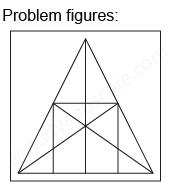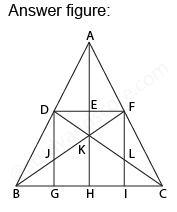## Practice Test Discussion

Q)

What is the number of straight lines and number of triangles in the given figure?• ExplanationAfter labelling the figure, we get the following,

• The horizontal lines are DF and BC (2)
• The vertical lines are DG, AH and FI (3)
• The slanting lines are AB, AC, BF and DC (4)

Total number of straight lines in the figure are 2 + 3 + 4= 9

Now, Counting the number of triangles,

• Simplest triangles are ADE, AEF, DEK, EFK, DJK, FLK, DJB, FLC, BJG, and LIC (10)
• Triangles with two components each ADF, AFK, DFK, ADK, DKB, FCK, BHK, KHC, DGB and FCI (10)
• Triangles with three components each DFJ and DFL (2)
• Triangles with four components each ABK, ACK, BFI, CDG, DFB, DFC and BKC (7)
• Triangles with six components each ABH, ACH, ABF, ACD, BFC, and CDB (6)
• Triangle with twelve components is ABC (1)

Total number of triangles in the figure is 10 + 10 + 2 + 7 + 6 + 1 = 36

Hence option C is correct.

- Share with your friends! -